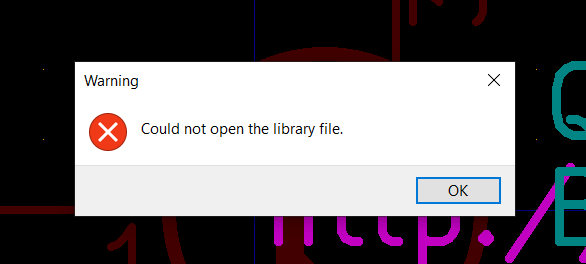# Copying derived symbols

I wanted to copy the BC847 symbol to my own lib for vendor ID code, but I got me this problem.

I understand what it means. I also found a workaround. I found out that it was derived from a BC817 and that one I saved it as a bc847 in my own library. Only after that I could change the fields with 817 into 847

This is sorta annoying. Is there an other workaround for copying derived symbols?

Regards,

Bas

Instead of saving the symbol have you tried exporting it to your own library? Seems to work for me. I get a BC847 added to my library without any parent.

2 Likes

as information: this missing feature is tracked in gitlab-issue 9740: Can't save derived symbol to other library (#9740) · Issues · KiCad / KiCad Source Code / kicad · GitLab

That issue was raised for v6 though. Don’t know whether it was addressed or not, didn’t go down the rabbit hole.

That “issue” has existed forever. If the symbol was in italics it could neither be “copied” nor “saved as”, even back in the days of 4.

Well, as I wrote, exporting the symbol to my own library worked for me, so maybe it got “fixed” as a result of other changes.

``````--- a.kicad_sym 2023-05-14 22:45:17.031951244 +1000
@@ -2916,6 +2916,86 @@
)
)
)
+  (symbol "BC847" (pin_names (offset 0) hide) (in_bom yes) (on_board yes)
+    (property "Reference" "Q" (at 5.08 1.905 0)
+      (effects (font (size 1.27 1.27)) (justify left))
+    )
+    (property "Value" "BC847" (at 5.08 0 0)
+      (effects (font (size 1.27 1.27)) (justify left))
+    )
+    (property "Footprint" "Package_TO_SOT_SMD:SOT-23" (at 5.08 -1.905 0)
+      (effects (font (size 1.27 1.27) italic) (justify left) hide)
+    )
+    (property "Datasheet" "http://www.infineon.com/dgdl/Infineon-BC847SERIES_BC848SERIES_BC849SERIES_BC850SERIES-DS-v01_01-en.pdf?fileId=db3a304314dca389011541d4630a1657" (at 0 0 0)
+      (effects (font (size 1.27 1.27)) (justify left) hide)
+    )
+    (property "ki_keywords" "NPN Small Signal Transistor" (at 0 0 0)
+      (effects (font (size 1.27 1.27)) hide)
+    )
+    (property "ki_description" "0.1A Ic, 45V Vce, NPN Transistor, SOT-23" (at 0 0 0)
+      (effects (font (size 1.27 1.27)) hide)
+    )
+    (property "ki_fp_filters" "SOT?23*" (at 0 0 0)
+      (effects (font (size 1.27 1.27)) hide)
+    )
+    (symbol "BC847_0_1"
+      (polyline
+        (pts
+          (xy 0.635 0.635)
+          (xy 2.54 2.54)
+        )
+        (stroke (width 0) (type default))
+        (fill (type none))
+      )
+      (polyline
+        (pts
+          (xy 0.635 -0.635)
+          (xy 2.54 -2.54)
+          (xy 2.54 -2.54)
+        )
+        (stroke (width 0) (type default))
+        (fill (type none))
+      )
+      (polyline
+        (pts
+          (xy 0.635 1.905)
+          (xy 0.635 -1.905)
+          (xy 0.635 -1.905)
+        )
+        (stroke (width 0.508) (type default))
+        (fill (type none))
+      )
+      (polyline
+        (pts
+          (xy 1.27 -1.778)
+          (xy 1.778 -1.27)
+          (xy 2.286 -2.286)
+          (xy 1.27 -1.778)
+          (xy 1.27 -1.778)
+        )
+        (stroke (width 0) (type default))
+        (fill (type outline))
+      )
+      (circle (center 1.27 0) (radius 2.8194)
+        (stroke (width 0.254) (type default))
+        (fill (type none))
+      )
+    )
+    (symbol "BC847_1_1"
+      (pin input line (at -5.08 0 0) (length 5.715)
+        (name "B" (effects (font (size 1.27 1.27))))
+        (number "1" (effects (font (size 1.27 1.27))))
+      )
+      (pin passive line (at 2.54 -5.08 90) (length 2.54)
+        (name "E" (effects (font (size 1.27 1.27))))
+        (number "2" (effects (font (size 1.27 1.27))))
+      )
+      (pin passive line (at 2.54 5.08 270) (length 2.54)
+        (name "C" (effects (font (size 1.27 1.27))))
+        (number "3" (effects (font (size 1.27 1.27))))
+      )
+    )
+  )
(symbol "Boost-converter" (pin_names (offset 1.016)) (in_bom yes) (on_board yes)
(property "Reference" "U" (at 0 -2.54 0)
(effects (font (size 1.27 1.27)))
``````

Is this observation symbolic of something?

Hi, we’re all busy discussing different functions.

The tally so far:
Copy - doesn’t work
Save As - doesn’t work
Export - does work.Question.

I was just looking. And it seems if I use the export option the symbol is going to be stored as it’s own library.

If I would like to store the transistor as a symbol inside any of my already existing libraries, would that be possible? I haven’t tried anything yet. Can you use import for that or something?

Regards,

Bas

Nope, I could store in my existing (personal, writable) library. Try again.

I was afraid of deleting all my hard work. Can you imagine?
I am gonna make a backup and try it outTry again

Well… I did. I hit export and selected one of my libraries.

Than this happened. The amount of luck I sometimes manage to find mehowcan?EDIT:
Despite the error the symbol is actually exported. So it does work

Bas

You have some kind of permission problem on the library file.